## Artigo

•Citado por SciELO
•Acessos

•Similares em SciELO

## versão impressa ISSN 1645-9911

### Tékhne  n.12 Barcelos dez. 2009

APAC: An exact algorithm for retrieving cycles and paths in all kinds of graphs

Ricardo Simões ,

Abstract. This paper presents an alorithm for retrieving all paths and all cycles between two vertices in random directed or undirected connected graphs. This algorithm can be easily implemented and is highly modular; with minor changes it can be adapted to obtain different parameters from the graphs. It is also demonstrated that the complexity of the algorithm increases linearly with the number of paths. The algorithm can be used in a myriad of applications. Aside from calculating all the paths and cycles in a graph, it can be used to calculate all the paths with length l between two vertices in the graph, as well as a solution to the clique decision problem. Thus, it has applications in computer networks, material science and electric networks, as well as in any problem where it is necessary to know the number of paths (not the optimal paths) in a directed or undirected connected graph or in multigraphs. The algorithms currently available in the literature, such as the depth-first search (DFS), are unable to solve this type of problems in a straightforward way.

Keywords: graph theory, connected graphs, random graphs; network paths, nanofiber networks

Resumo. Este artigo apresenta um algoritmo para identificar todos os caminhos e todos os ciclos entre dois vértices em grafos conexos aleatórios (orientados ou não orientados). O algoritmo pode ser facilmente implementado e é altamente modular; com pequenas alterações pode ser adaptado para obter diferentes parâmetros a partir dos grafos. Também demonstramos que a complexidade do algoritmo aumenta linearmente com o número de caminhos.

Este algoritmo pode ser utilizado num vasto número de aplicações. Para além de calcular todos os caminhos e ciclos num grafo, pode também ser utilizado para calcular todos os caminhos com comprimento l entre dois vértices do grafo, assim como solucionar o problema de decisão clique. Assim, tem aplicações em redes de computadores, ciência de materiais e redes eléctricas, assim como qualquer problema em que seja necessário saber o número de caminhos (não o caminho óptimo) num grafo conexos (orientados ou não orientados) ou multi-grafos. Os algoritmos actualmente disponíveis na literatura, como o depth-first search (DFS), não conseguem resolver este tipo de problema de forma simples.

Palavras-chave: teoria de grafos, grafos conexos, grafos aleatórios, redes, nanofibras

1. Introduction

This article focuses on the problem of finding all the paths and all the cycles between two vertices in random directed or undirected connected graphs. This information can be used, for example, as a metric in several situations that arise in random network problems.

Finding all paths on a graph implies finding the longest simple path in the graph. As the later is a NP-Complete problem, the overall problem is thus NP-Complete (Berman & Schnitger, 1989; Cormen, 2005). With this in mind, a simple and highly modular algorithm was developed, which was termed APAC (All Paths And Cycles), and which can be used in all types of relatively dense graphs.

A typical solution for finding the shortest paths between all vertices in an unweighted graph is running a breadth-first search for all vertices in the graph. Another type of problem that is closer to the problem of finding all paths and cycles in a graph, is the k-shortest paths one. This optimization problem focuses on finding the k best solutions between two vertices. There is extensive literature for this kind of problem, with several algorithms that can solve the problem; (Brander & Sinclair, 1995; Eppstein, 1999; Hershberger et at, 2007) and their references are a good starting point.

The main difficulty in using some instance of k-shortest paths algorithms in the current problem is that they are based in an optimization problem, and most of them only work for specific types of graphs (directed or undirected). Aside from that, they rely on sophisticated data structures, which makes them far from trivial to implement. Yet another constrain is that the definition of paths varies from algorithm to algorithm; whereas some find the k-shortest paths with repeated vertices, others define the paths without repeated vertices.

The intention here is to apply the developed APAC algorithm to less dense subgraphs that emerge from a random network (Barabasi & Albert, 1999), graphs where the number of edges is lower than in a complete graph. A priori, the number of paths is known to be small. Therefore, an algorithm that scales linearly with the number of the paths in a graph is appropriate for the type of problems describe above.

Aside from the obvious application of identifying the paths and cycles in a graph, this paper also indicates two other possible applications of this algorithm:

· Generation of one of the required inputs for the use of a known graph distance metric (Horst & Kim, 1998), based on the maximum common subgraph (MCS) of two graphs.

· Study the variation of the number of paths in response to parameters of a physical system (namely in this case, the network complexity).

2. Notation

Throughout this article, the book of Cormen (2005) is used for the terminology and notation not defined here.

Definition 1. The set of all vertices (νi) is V. The set of all edges (eij) is E.

Definition 2. A digraph (G) is a finite collection of vertices and directed edges (eij) joining certain pairs of vertices, with G = (V, E).

Definition 3. A graph (G) is a finite collection of vertices ν1, ν2, ν3...,νn and undirected edges  eij=eji joining certain pairs of vertices, with G = (V, E).

Definition 4. A path (P) between vertices is a sequence of edges (with no repeated vertices) that allows one to proceed in a continuous manner from one vertex to another. The length of a path is its number of edges.

Definition 5. A cycle (C) is a closed path (P). This is, a path where the initial vertex (ν0) is equal to the final vertex (νn).

Definition 6. The degree (d) of a vertex (d(νi)) in an undirected graph is the number of edges incident to (ν).

Definition 7. Let G, G1, and G2 be graphs. G is a common subgraph of G1 and G2 if there exists subgraph isomorphisms from G to G1 and from G to G2; definition 5 in (Horst & Kim, 1998).

Definition 8. A common subgraph G of G1 and G2 is maximal if there exists no other common subgraph G' of G1 and G2 that has more nodes than G; definition 6 in (Horst & Kim, 1998).

Definition 9. from (Horst & Kim, 1998): The distance of two non-empty graphs G1, G2 is defined as: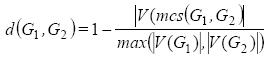Where MCS is defined as the maximum common subgraph of two graphs, and |V(G)| is the number of nodes of the graph G.

Definition 10. The average degree of a graph is given by: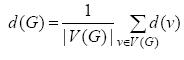Theorem 1. from (Horst & Kim, 1998): For any graphs G1, G2 and G3, the following properties hold true:

1. 0≤ d(G1, G2) ≤1

2. d(G1, G2)=0, which implies that G1 and G2 are isomorphic to each other.

3. d(G1, G2) = d(G2, G1)

4. d(G1, G3)d(G1, G2) + d(G2, G3)

Proof in (Horst & Kim, 1998).

3. Related work

In a pioneer paper, Lars-Erik (1996) developed an algorithm that solves the problem of finding all paths, as a reachability matrix, in a directed graph. That algorithm is suitable if the number of edges is lower than the number of nodes. It uses an incidence matrix (A) as an input and outputs the reachability matrix (B). To build the reachability matrix the author represents A as a list of all edges or pair of nodes and uses the known equation B = (A + I) n-1, where I is the unit matrix. The algorithm selects a pair of vertices {νa}, {νb} ∈ V(G) and verifies whether {eab} ∈ E(G); applied sequentially, this enables the reachability matrix to be built. The first steps of our algorithm are based in this initial work; however, the present work is not looking for the reachability matrix but for all simple paths between any two given vertices.

Horst and Kim (1998) introduced a new distance metric based on the topology of the graph and formally proved it. The previous mentioned metric is based in the MCS, which is also a NP-Complete problem (Berman & Schnitger, 1989; Gary & Johnson, 1979). One needs two less dense graphs to use the metric in an efficient way. To circumvent this problem one can construct from the initial graph (G) a subgraph (G1) using the k-shortest paths of G. The second induced subgraph (G2) is constructed by retrieving all the paths in the initial graph, after the application of an edge-deletion algorithm (similar to the algorithms described in (Albert et al, 2000; Broder et al, 2000, Farid & Christensen, 2006). Thus, using the previously described procedure, the MCS based metric can be applied to two graphs in a relatively fast way. This is possible because one can control the density of G1 (by varying the k-shortest paths) and it is known that the induced subgraph (G2) has a number of edges higher than the vertices but lower than a complete graph.

In the context of the k-shortest problem (simple paths between a single origin and a single destination), Brander and Sinclair (1995) selected and studied, from more than seventy papers, four algorithms [Yen, 1971; Lawler, 1972; Katoh et al, 1982; Hoffman & Pavley, 1959). These were chosen by their expected speed of operation in undirected graphs, with no parallel edges, no self-loops and no negative edges. The numerical study shows that the Lawler (1972) algorithm, with O (kn3) for the computational complexity, had the best result. Other references for the same problem can be found in (Eppstein, 1999; Hershberger et al, 2007).

For the problem of finding the maximum common subgraph, the work by Bunke et al (2002) provides a comparative study between a space search based algorithm, and one based on clique detection performed on randomly connected graphs. As a result of this study, the authors conclude that for low density graphs the best algorithm is the space based one.

4. APAC algorithm

In the design of this algorithm, it is important to keep in mind the issue of solving a NP – Complete problem. Thus, let us set a limit to less dense connected graphs, meaning, graphs where the number of edges is larger than the number of vertices but lower than in a complete graph. Another important aspect is the types of graphs where this algorithm is planned to be implemented. The graphs can be undirected, directed, or even graphs with parallel edges (multigraphs). Finally the algorithm must be sufficiently modular; with simple modifications, one can explore other attributes of the paths without having to rewrite large parts of code or the need to employ a different algorithm.

The depth-first search (DFS) approach resembles this algorithm, but as can be seen in the following sections, the APAC algorithm does not need to keep track of all visited vertices, and only stores the feasible paths. Thus, the data structures used by the DFS to keep track of the discovered vertices are not present in the APAC algorithm. Another important difference is the range of application of the two algorithms, which is quite different. The APAC algorithm retrieves all paths in a graph (and the respective cycles), while the DFS is used to transverse directed and undirected graphs but does not retrieve all paths in a graph. Due to that, the DFS is typically used as a subroutine in other algorithms (Cormen, 2005).

4.1 Description

In this section are presented some definitions required to understand the APAC algorithm pseudo-code shown in Figure 1.

· PUSH: This a Stack function; typically adds one object to the stack.

· STACK-EMPTY: This is a Stack function; returns true if is empty.

· TAIL: This set function returns the last vertex.

· POP: Removes the element at the top of the stack.

· FIND-NODE: Returns true if an existing vertex already exists in a given set.

· OUT-PATH: Return a found path to some data structure or write it to disk.

· OUT-CYCLE: Return a found cycle to some data structure or write it to disk.

· {s}: Is the initial vertex.

· {t}: Is the final vertex.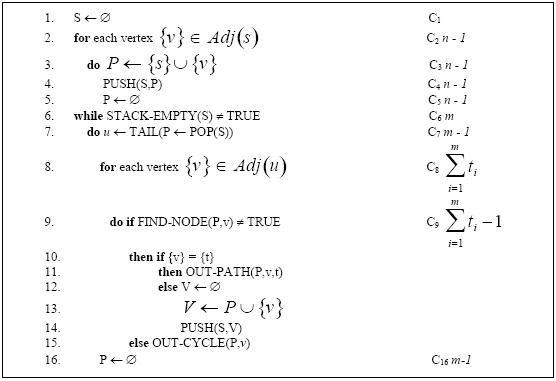Figure 1. APAC algorithm

4.2 Analysis of the algorithm

Lemma 1. Each call to the function Out-Path constructs a unique path between (νm, νn).

Proof. Suppose that the claim is true for some undirected graph (G) with no self-loops.

From a starting vertex {s} one can construct (n-1) unique sets P (line 2), from the fact that these sets are the edges incident to {s}, and that imposed condition of no self-loops in the graph.

Then, from line (8) in the algorithm, it is known that {ν} ∈ Adj[u] which implies that (u, ν) ∈ E(G). From line (9) it is imposed that there are no repeated vertices in the set P. If the condition in line (9) and (10) is fulfilled by the definition of a path (definition 4), (u,v) is a path. The set P is also unique because, at each step of the algorithm, either the the (s,t) path is found or the set P is incremented with another edge (line 13). As the added edges of the graph are unique (there are no repeated vertices), then P is unique.

Theorem 2. Let G be an undirected or directed connected graph with no self-loops.

It is claimed that:

(a)     The algorithm finds all simple paths.

(b)     The algorithm finds all cycles.

(c)     The algorithm terminates.

(d)     The algorithm complexity is O (Cnpaths).

Proof. From Lemma 1, it is known that the set of edges (paths) retrieved in line (11) is unique. Suppose that for some undirected connected graph G and for any given iteration of the algorithm there exists a set P (sequence of vertices) such that all vertices that belong to P are unique. Finally suppose that the condition in line (9) is always fulfilled. For all {ν} ∈ Adj[u] in line (8), if {ν}={t} then by Lemma 1 Out-Path generates a unique path, otherwise if {ν}≠{t} the set P=P∪{ν} is moved to the stack. In the later case, as this is a last in first out (LIFO) procedure, that set P will be analyzed in the next iteration. If the for in line (8) is not called then this means Adj[u] = Ø, and the P set is cleared by the instruction in line (16). If at this point the stack is also empty, the algorithm terminates by the instruction in line (6), proving (c).

For each set P retrieved from the stack in line (7), the instruction in line (10) will be tested for w vertices associated to that specific set. According to line (10), if one of those vertices is {t}, then a unique path has been found according to Lemma 1. Since this occurs n times, for every set P in which one of the w vertices is equal to {t}, all the n unique paths in the graph are found, proving (a). Continuing with the last argument, and supposing that parameters are imposed such that the condition in line (9) is not fulfilled, then the Out-Cycle is called producing a cycle with length l, which proves (b).

Let T(m) be the maximum possible computational execution time for the APAC algorithm. Here, m is the maximum number of iterations of the while cycle of line (6), Cinit is the computational execution cost associated to the first 5 lines, and C9 is the overall cost in the execution of line (9). The number of iterations in line (8) can be approximated to <d(G)>, where <d(G)> is the average degree of the graph. On average, npaths is the average path length multiplied by the number of paths. Based on these assumptions, Equation 1 shows the derivation of the execution time of the APAC algorithm.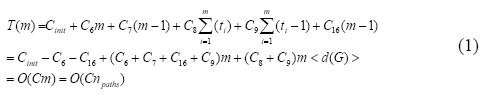As can be seen, the algorithm scales linearly with the number of paths, assuming that the number of paths is very large with respect to the average degree, proving (d).

The algorithm was tested using the model proposed by Erdos and Renyi (1959) for generating a random graph. In their pioneer work, they study the distribution of the minimum and maximum degree in a random graph.

They generate a random graph by initially defining a maximum number of edges and, from an initial set of disconnected vertices, randomly picking one pair of vertices and forming an edge.

A similar procedure was followed for generating the graphs (undirected). In this method, the number of vertices was fixed to 10, and 10000 connected graphs were generated with a random number of edges. The maximum number of edges was set to that of a complete graph.

For each generated graph, the APAC algorithm was applied and it was found that the time complexity agrees with the expected values; see Figure 2.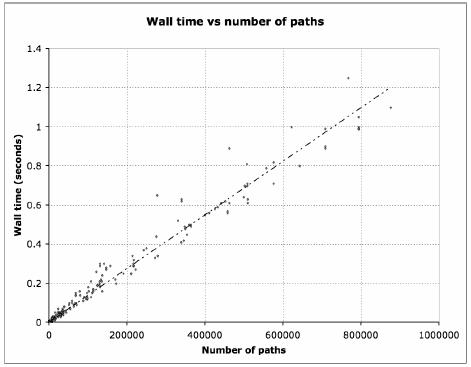Figure 2.  Wall time vs number of paths

4.3 Modularization examples

If one uses a queue instead of a stack then a first in first out (FIFO) policy is obtained. With this simple modification, one can change the way paths appear in the output.

Let us turn our attention to the graph represented in Figure 3. By applying the APAC algorithm to this graph, the stack has two initial sets, {s,A} ,{s,C}. Observing Figure 3, the maximum length of a path in the graph can be easily seen to be three and the minimum length to be two.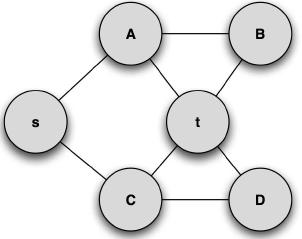Figure 3. Example undirected Graph

Using the stack as a dynamic set, the order of the paths will be {s,C,t},{s,C,D,t},{s,A,t},{s,A,B,t}. If instead a queue is used the order of paths will be {s,C,t},{s,A,t},{s,C,D,t},{s,A,B,t}

Thus, with this simple change, the order of appearance of paths is changed.

Another modification that can be used (using a queue) is adding another restriction in line 6. If the length of the set is higher than some threshold, then stop the while cycle. With the previous modification the algorithm only retrieves the paths of length k, which enables easy finding of k-shortest paths (with no costs associated in the edges). Using the stack procedure ensures that every possible path is exhausted from an initial branch of the graph.

4.4 Implementation considerations

In practical terms, it should be pointed out that the initial graph is stored in an adjacency list type data structure and the paths and the cycles are stored as sequences of edges in some user defined data structure. The algorithm was implemented using the data structures provided by the C++ standard template library. The study shown in Figure 2 was generated in a laptop computer with a core2 duo intel processor, and the code was compiled using the GNU C++ complier.

The algorithm presented in Figure 4 is the one implemented. One can easily see that it is the same algorithm presented in section 4.1 and analyzed in section 4.2. Note that in section 4.2 it was necessary to move the initial iteration out the while cycle, only to facilitate the analysis of the algorithm. The time bounds are not affected by this small change.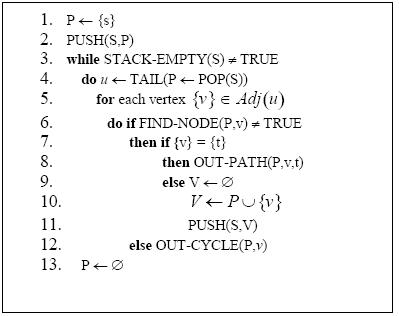Figure 4. The implemented algorithm

5. Application Examples

In this section two of the multiple applications of the algorithm are described.

5.1 Graph distance metric

Let us consider the initial undirected graph represented in Figure 5.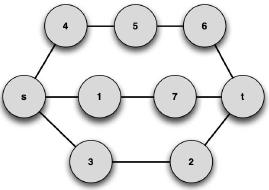Figure 5. Initial graph (G).

For this graph, the previously described MCS-based metric (Horst & Kim, 1998) will be applied. For this purpose, the version of APAC with the queue instead of the stack will be employed, and a prescribed path length of 3 edges is imposed (the minimum value in this graph). The k-shortest path subgraph G1 is represented in Figure 6.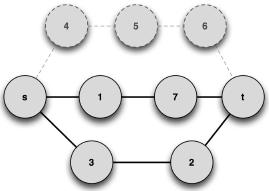Figure 6. Subgraph G1. Solid lines and nodes represent edges of the subgraph; dashed lines and nodes represent edges from the initial graph (shown only for visualization purposes).

The previous subgraph was built with paths of length k retrieved from the graph represented in Figure 5. Now using an edge-deletion algorithm, specific paths can be retrieved from the initial graph, namely {s,4,5,6,7,t} and {s,1,7,t}, from which graph G2 is built; see Figure 7. Note that this graph is also a subgraph of G.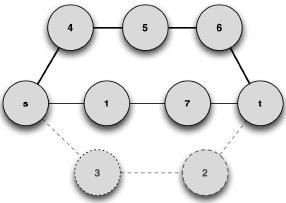Figure 7. G2 induced graph, by some deleted edge algorithm. Solid lines and nodes represent edges of the subgraph; dashed lines and nodes represent edges from the initial graph (shown only for visualization purposes)

Using Definition 9, a possible maximum common subgraph can be found (note that the MCS is not unique), as shown in Figure 8.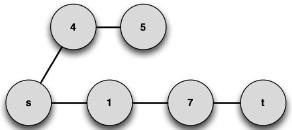Figure 8. The maximum common subgraph of G2 and G1.

Applying the distance metric to G1 and G2, one can find d(G1, G2) = 0.14. Using the previous metric, it is possible to find the distance between two graphs, or the degree of isomorphism that exists between the two graphs (the lower the distance, the more isomorphic the graphs are). This can be a very important aspect in solving problems under some specific contexts.

5.2 Study on the effect of the average degree

One can also apply the APAC algorithm to random (undirected) graphs generated from randomly positioned particles in a 3D volume. By considering an increasing range for interactions between particles (in small steps), the average degree is increased. For each range of interactions, an edge-deletion algorithm is applied (Albert, 2000; Broder, 2000; Farid & Christensen, 2006), giving rise to an induced subgraph. One can then employ the APAC algorithm to both the initial graph and the induced subgraph; this is then repeated for each average degree. The result is shown in Figure 9.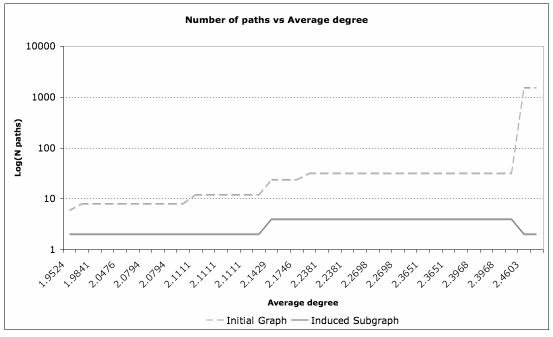Figure 9. Variation of the average degree versus the number of paths.

It was found that the number of paths increases substantially at specific average degrees, due to specific spatial arrangements of the particles. However, it was also observed that this does not influence the number of paths in the induced subgraph (that results from the edge-deletion algorithm), which indicates that a higher range of interactions does not seem to influence the paths relevant for the applied edge-deletion algorithm.

6. Concluding Remarks

A simple and highly modular algorithm, termed APAC, was developed to identify all paths in lower dense graphs, which is a NP-Complete problem.

Traditional k-shortest paths algorithms are not suited for finding the total number of paths between two nodes in a graph. They are designed for optimization problems, for specific types of graphs, and include several other constrains. Using APAC, it is possible to find which edges those paths are comprised of. Moreover, this search can be performed on all types of graphs. Since the edges belonging to each path are known, the length of different paths can be compared, and a subgraph can also be built with these edges. The ability to build these subgraphs is a valuable resource, since it allows characterizing topological features of the system under study.

The APAC algorithm used in the context of a distance graph metric (Horst & Kim, 1998) can provide valuable information for different problems, both those related to physical as well as information systems. This metric could be applied, for example, to the problem of dielectric breakdown (Beale & Duxbury, 1988; Gyure & Beale, 1992), answering some unsolved questions related to minimum shortest paths and breakdown paths. The algorithm can be easily adapted to deploy other metrics, which can be valued as they may relate to important properties of the system or to its behavior.

This algorithm can be a useful tool for complex system analysis. In this framework, a clear example of a potential application would be information networks, such as the publications citation network and the world wide web.

Acknowledgements

The author would like to thank the contribution of Jaime Silva (University of Minho, Portugal) to the development and coding of the APAC algorithm, and Richard Vaia (Air Force Research Laboratories, USA) for providing the original challenge of developing improved methods to predict the electrical properties of nanocomposites, and to acknowledge the Foundation for Science and Technology, Lisbon, through the 3° Quadro Comunitario de Apoio, the POCTI and FEDER programs.

Referências

Albert, R., Jeong, H., & Barabási, A. L. (2000). Error and attack tolerance in complex networks, Nature 406, 378.        [ Links ]

Barabasi, A. L., & Albert, R. (1999). Emergence of scaling in random networks, Science 286, 509-512.

Beale, P. D., & Duxbury, P. M. (1988). Theory of dielectric breakdown in metal-loaded dielectrics, Phys. Rev. B 37, 2785-2791.

Berman, P., & Schnitger, G. (1989). On the complexity of approximating the independent set problem, Proc. of the 6th Annual Sym. on Theoretical Aspects of Computer Science on STACS 89, Paderborn, Germany, 256-268.

Brander, A. W., & Sinclair, M. C. (1995). A comparative study of k-shortest path algorithms, Proc. 11th UK Performance Engineering Worksh. for Computer and Telecommunications Systems, Liverpool, 370-379.

Broder, A., et al (2000). Computer Networks 33, 309-320.

Bunke, H., Foggia, P., Guidobaldi, C., Guidobaldi, C., Sansone, C., & Vento, M. (2002). A Comparison of algorithms for maximum common subgraph on randomly connected graphs, Structural, Syntactic, and Statistical Pattern Recognition, Springer, 85-106.

Cormen, T. T., Leiserson, C. E., & Rivest, R. L. (2005). Introduction to algorithms, second edition, MIT Press, Cambridge MA USA.

Eppstein, D. (1999) Finding the k shortest paths, SIAM J. Comput. 28, 652-673.

Erdos, P., & Reny, A. (1959). On random graphs, Publicationes Mathematicae 6, 290–297.

Farid, N., & Christensen, K. (2006). New J. Phys. 8, 212-229.

Gary, M. R., & Johnson, D. S. (1979). Computers and intractability: a guide to the theory of np-completeness, WH. Freeman and Co, New York NY USA.

Gyure, M. F., & Beale, P. D. (1992). Dielectric Breakdown in continuous models of metal-loaded dielectrics, Phys. Rev. B 46, 3736-3746.

Hershberger, J., Suri, S., & Bhosle, A. (2007). On the difficulty of some shortest path problems, ACM Trans. Algorithms 3, No. 5.

Hoffman, W., & Pavley, R. (1959). Method for the solution of the nth best path problem, Journal of the Association for Computing Machinary 6, 506-514.

Horst, B., Kim, S. (1998). A Graph distance metric based on the maximal common subgraph, Pattern Recognition Letters 19, 255-259.

Katoh, N., Ibaraki, T., & Mine, H. (1982). An efficient algorithm for the k shortest simple paths, Networks 12, 411-427.

Lars-Erik, T. (1996). An algorithm for computing all paths in a graph, BIT 6, 347-349.

Lawler, E. L. (1972). A procedure for computing the k best solutions to discrete optimization problems and its applications to the shortest path problem, Management Science Theory Series 18, 401-405.

Yen, J. Y. (1971). Finding the k-shortest loopless paths in a network, Management Science 17, 712-716.

Brief CV

Ricardo Simoes is an Associate Professor at the Polytechnic Institute of Cávado and Ave, Portugal. He conducts research activities at the Institute for Polymers and Composites of the University of Minho, and the Associate Laboratory Institute of Nanostructures, Nanomodelling and Nanofabrication (I3N). He cooperates with the MIT-Portugal program (Engineering Design and Advanced Manufacturing area).

He has a 5-year bachelor degree in Polymer Engineering from the University of Minho and a PhD in Materials Science and Engineering from the University of North Texas, USA. He is currently coordinating projects in mobile health (MIT-Portugal program), RFID (SimeI&DT), automotive (with Ford Motor Company), nanomaterials (with the US Air Force Research Labs), and medical devices (with Health Cluster Portugal). He has over 40 scientific/technical publications, over 20 oral presentations at international conferences, and 8 invited lectures in several countries.

 Polytechnic Institute of Cávado and Ave, Campus do IPCA, 4750-810 Barcelos, Portugal; rsimoes@ipca.pt

 IPC – Institute for Polymers and Composites, University of Minho, Campus de Azurém, 4800-058 Guimarães, Portugal; rsimoes@dep.uminho.pt